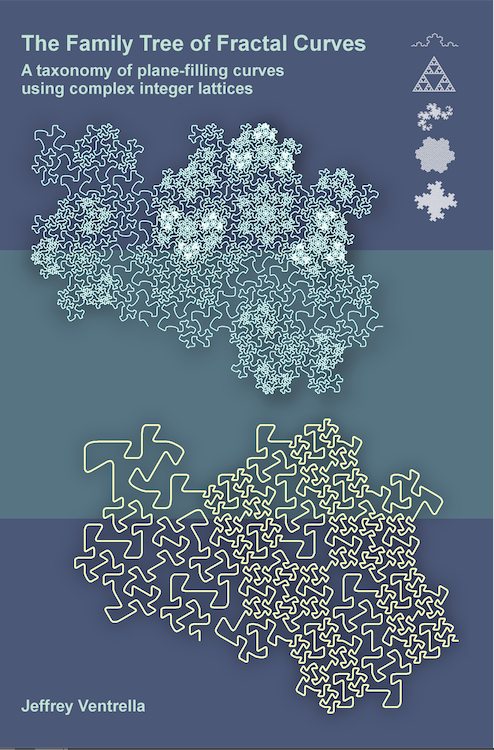The Family Tree of Fractal Curves A taxonomy of plane-filling curves using complex integer lattices by Jeffrey Ventrella 1 Introduction 2 Taxonomy 3 Iteration 4 Morphology 5 Family Resemblances 6 Undiscovered Curiosities 7 References

 3. Iteration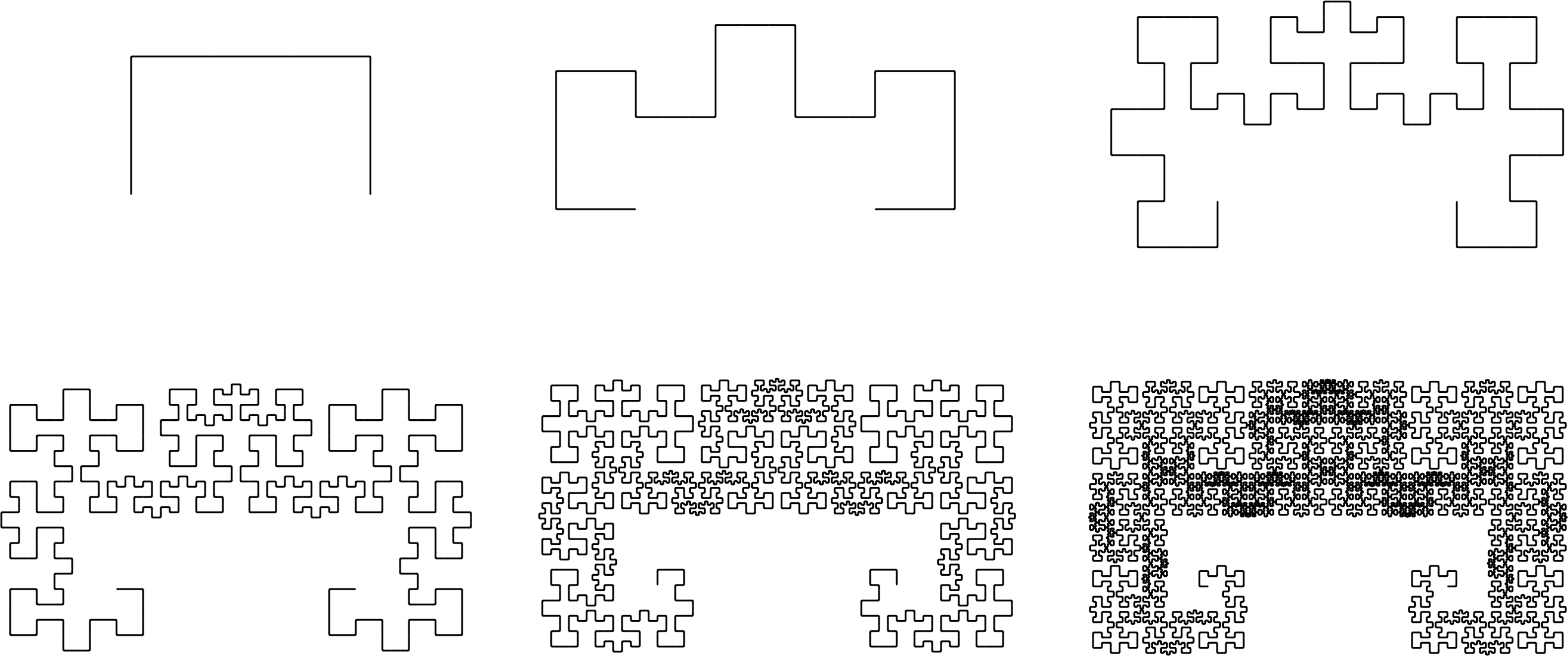Figure 3.1. A self-avoiding curve of the E(3,0) family A key thesis in this book is that the complex integers (G and E) can be used as a framework to analyze and classify edge-replacement plane-filling curves. But complex integers can also be used in the process of generating these curves. As an example, let’s explore a technique for creating teragon 2 of the 5-Dragon. It is a member of the G(2,1) family and its norm is 5. It requires no transforms. Figure 3.2. shows the five ordered Gaussian integers of its generator at the top. Teragon 2 can be generated by multiplying five copies of the generator by its own integers, and summing them.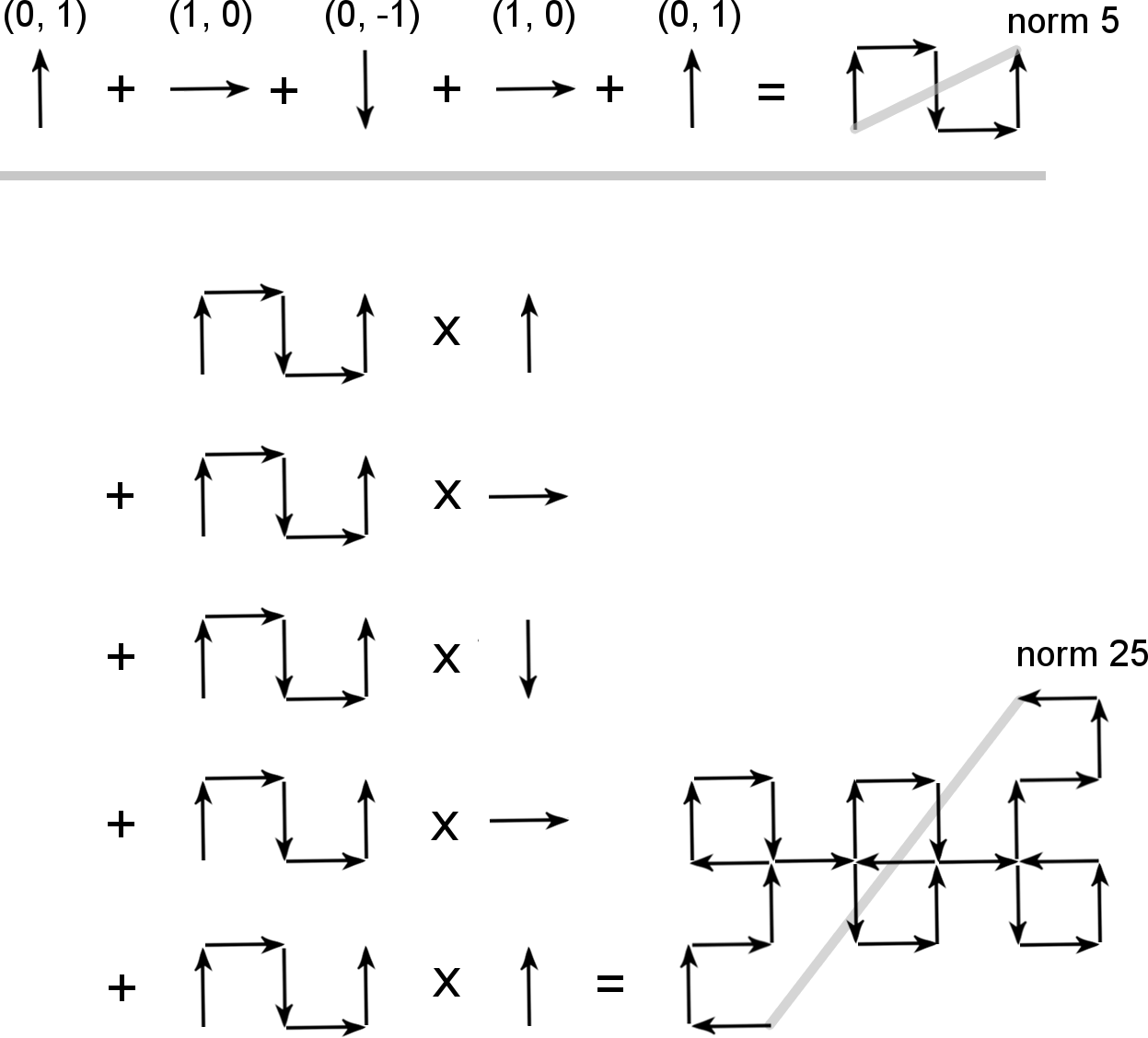Figure 3.2. Multiplying the 5-dragon generator with itself The endpoint of the curve (f) is raised exponentially for each teragon order, as shown in Figure 3.3Figure 3.3. Rotation and dilation of 5-Dragon generator As iteration progresses, the teragons rotate and dilate along the curve of the G(2,1)n family set, as shown in Figure 3.4.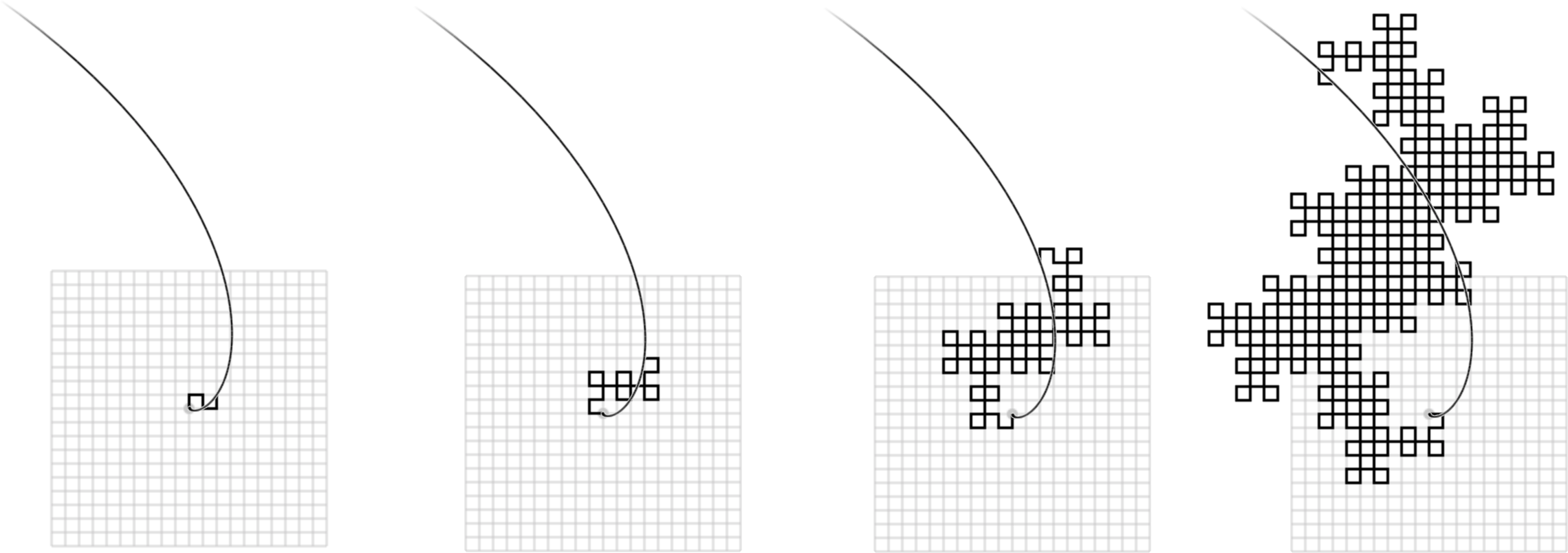Figure 3.4. As the 5-Dragon iterates, it rotates and dilates along the G(2,1)n spiral Figure 3.5 is similar; it shows how teragons of the HH Dragon undergo rotation and dilation, with their endpoints following the power-of-2 spiral with each iteration. Below are three high-order teragons, used to illustrate rotation and dilation.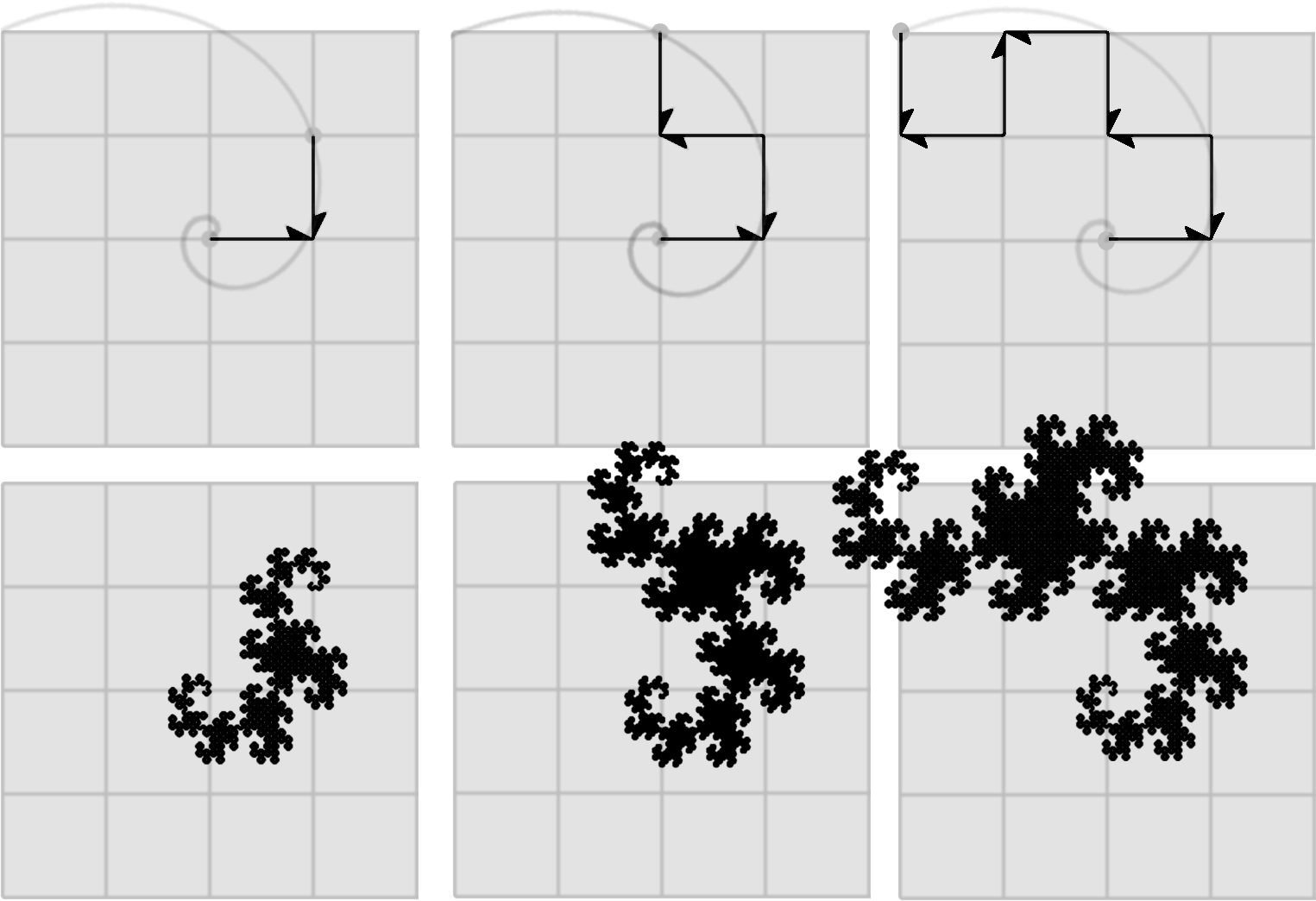Figure 3.5. Each progressive teragon follows a rotation and a dilation. Figure 3.6 illustrates a process for generating the first four teragons of a curve from the G(2,2) family, called “Brainfiller” .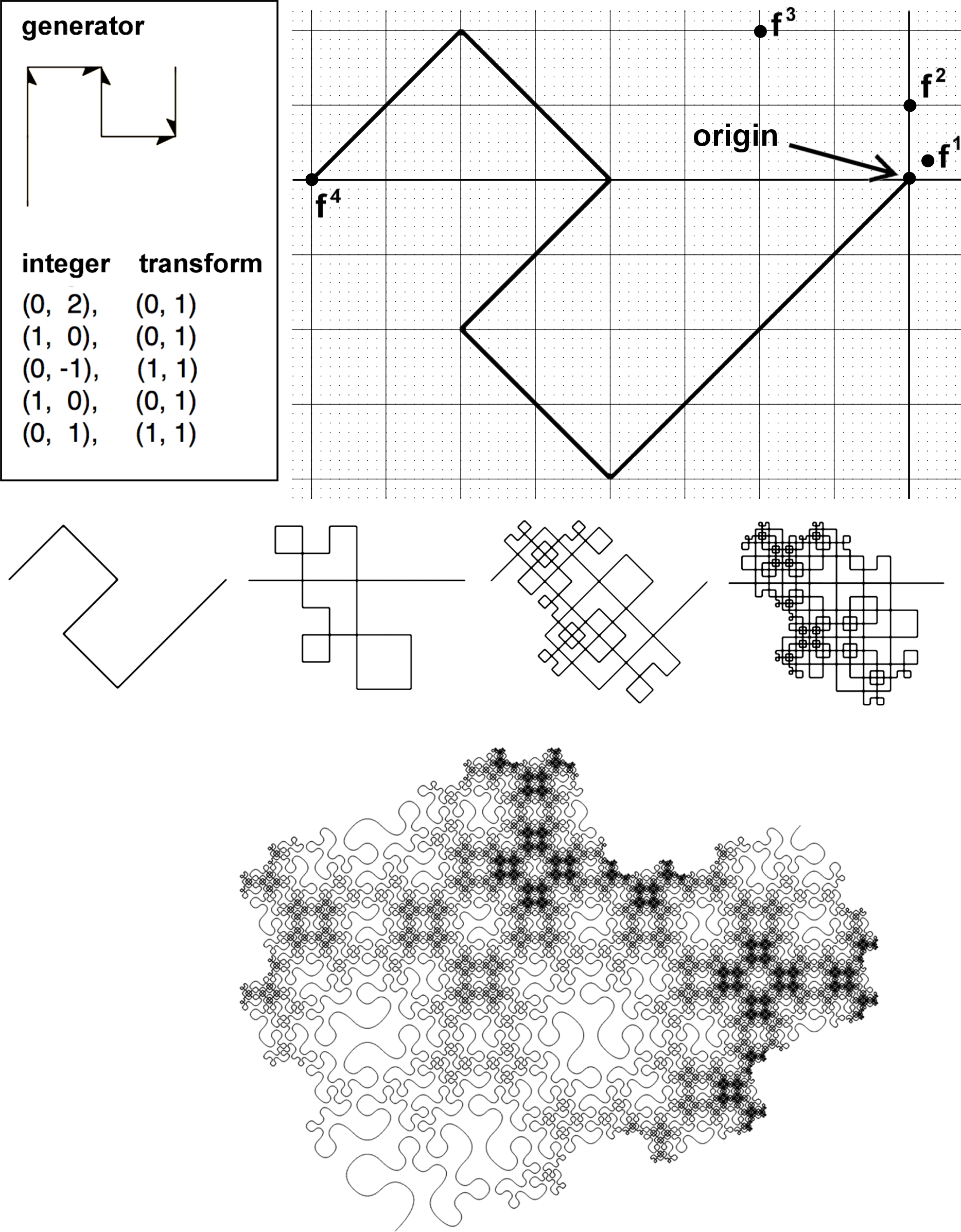Figure 3.6. Iteration of the Brainfiller curve The generator for the Brainfiller curve is shown at top-left of Figure 3.6. The family integer G(2,2) has norm 8; it is shown at the far-right as f1. Raising this integer to the power of 4 (the teragon order) results in the integer G(-64,0), which lies on the power-of-8 spiral. It has norm 4096 and is shown as f4. Multiplying the generator by f3 results in a dilated and rotated copy of the generator, whose endpoint is f4. With the set of integers lying along the G(2,2) spiral, all possible transforms of the generator can be placed on any segment and the vertices will conform to integer points on the lattice. A recursive function is used to generate the integers of the curve. Transform state is carried through the recursive steps, determining how the generator is transformed at each step. When recursion is complete, the smallest integer norm in the curve is 1. The teragons of this curve are partially self-contacting. Teragon 5 is shown as a rotated, scaled, and splined rendering at the bottom of the figure. Transforming back to Family Space Since this complex number-based technique causes rotation and dilation, computing a teragon of a high order puts the endpoint at a large distance from the origin, with a rotation that may change for each consecutive teragon. The integers can be transformed back to the space of the original generator—to normalize the teragon for purposes of visualization. Essentially, this technique pulls the geometry out of the integer lattice and brings it into the full complex plane, where it can be multiplied arbitrarily. The majority of the examples in this book showing multiple teragons of a single curve have been transformed to occupy the space of the family interval. This transformation allows every curve—regardless of its teragon order (and thus size and rotation)—to be viewed in the family space, as shown with the first six teragons of the 5-Dragon in Figure 3.7.Figure 3.7. Teragons 1-6 of the 5-dragon (scaled to the family integer) Integer Genetics Composite integers have a trivial kind of of self-similarity. For instance, the rational integer 35 can be described as 7 similar 5’s (or 5 similar 7’s). Highly-composite numbers, such as 60, have more degrees of self-similarity. But only perfect powers have scaling self-similarity, making them fractal-like. Consider the rational integer 81, which can be expressed as 34. The self-similarity of this perfect power is shown as a stylized partitioning in Figure 3.8.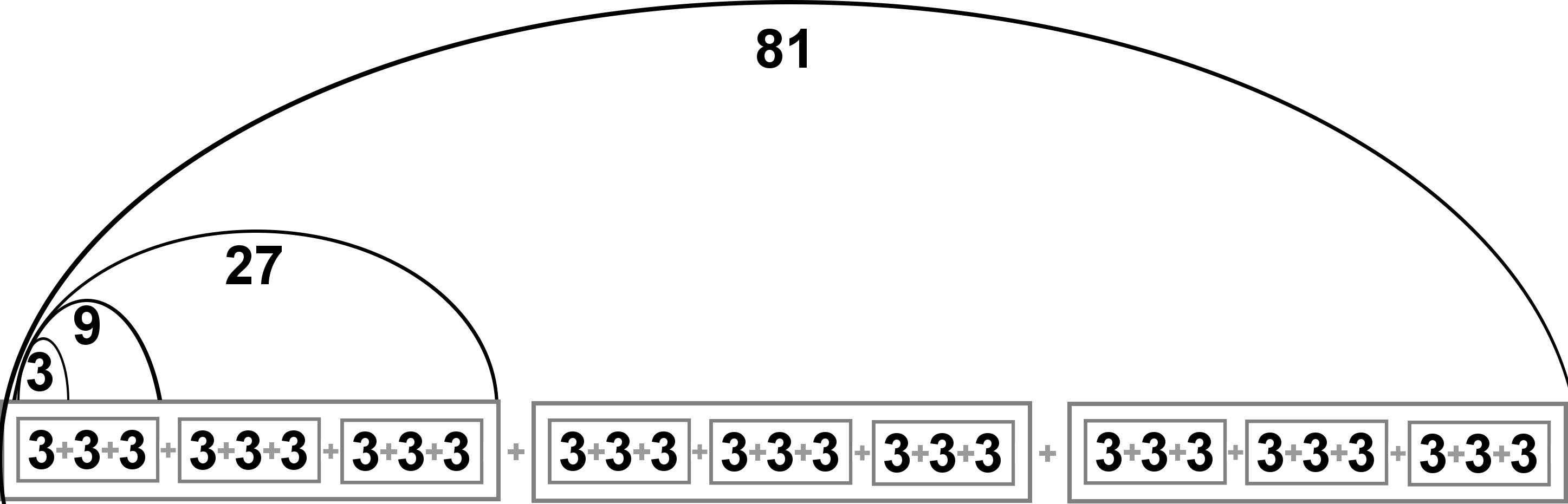Figure 3.8. Self-similarity of the rational integer 81 as 34 The self-similarity of the integer 81 is also expressed in the E(2,1)n family set (Terdragon teragons 1 through 4) shown in Figure 3.9.Figure 3.9. Self-similarity of the Eisenstein integers with norms 3, 9, 27, and 81 Integer divisibility and exponentiation are key concepts in this curve-generating technique, and the taxonomy upon which it is based. It is distinct from other kinds of algorithms for computing fractal curves, such as the use of turtle geometry controlled by an L-system. Turtle geometry uses polar coordinates (angles and lengths). Depending on the curve, the generator’s angles and lengths might be real numbers; they may even be irrational—in which case, it is impossible to specify them with full numerical precision. But when the entire specification of the curve is in terms of complex integers (by connecting the points in a lattice), the data footprint becomes relatively small, and computation is more precise, as shown in Figure 3.10.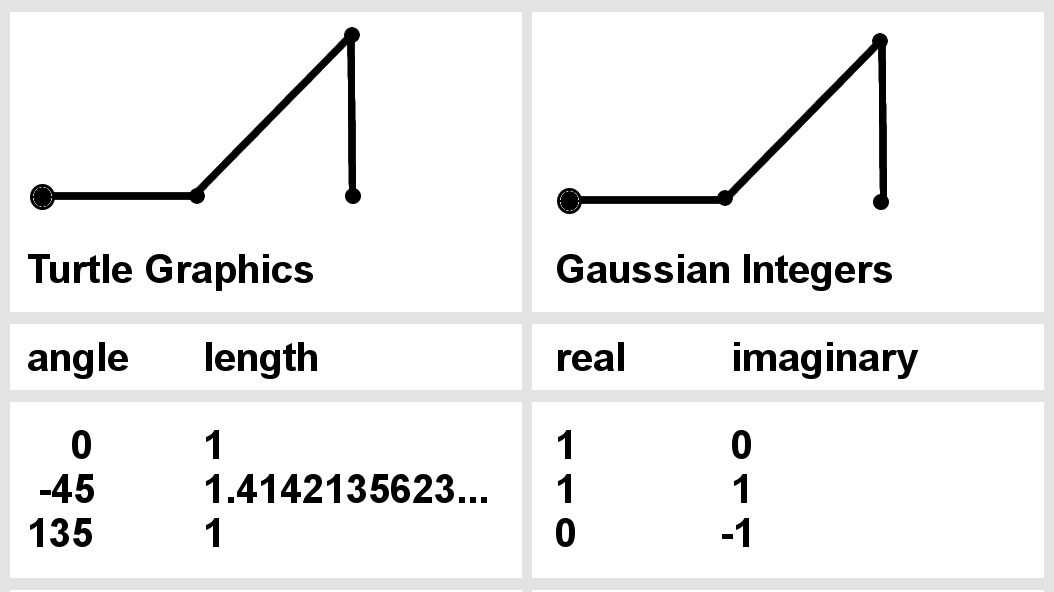Figure 3.10. Comparing data footprint of turtle geometry vs. integer-based scheme Reducing the genetic specification of a curve to a small integer representation is an attempt at representing curves using the most fundamental, irreducible distinguishers among them. This technique is an exercise in efficient encoding. This genetic encoding—when reduced to the smallest possible expression—consists of a single bit to specify the domain (G vs. E), an array of complex integers, and an array of transform pairs (each of which is one bit). With this encoding, the family integer could be left out, since it can be derived from summing the integer array. Figure 3.11 shows an example of this minimal genetic encoding for a curve in the E(2,0) family.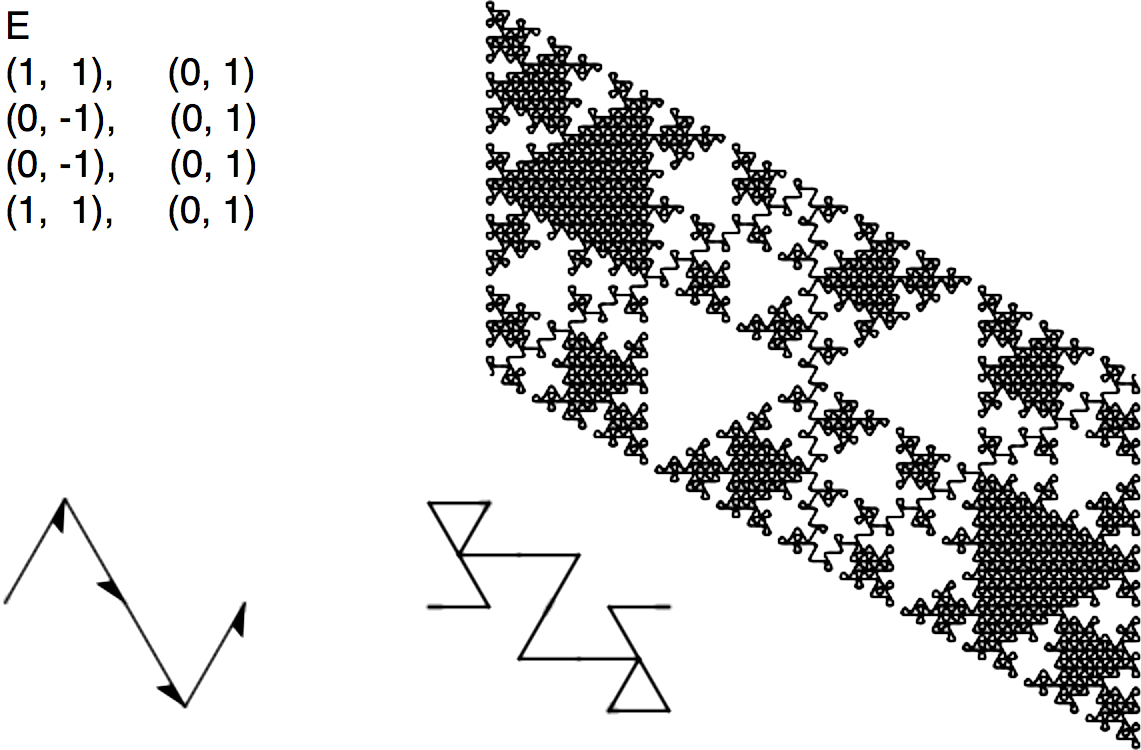Figure 3.11. A curve of the E(2,0) family, showing minimal genetic encoding Figure 3.12 shows Mandelbrot’s Snowflake sweep, specified with this abbreviated genetic encoding.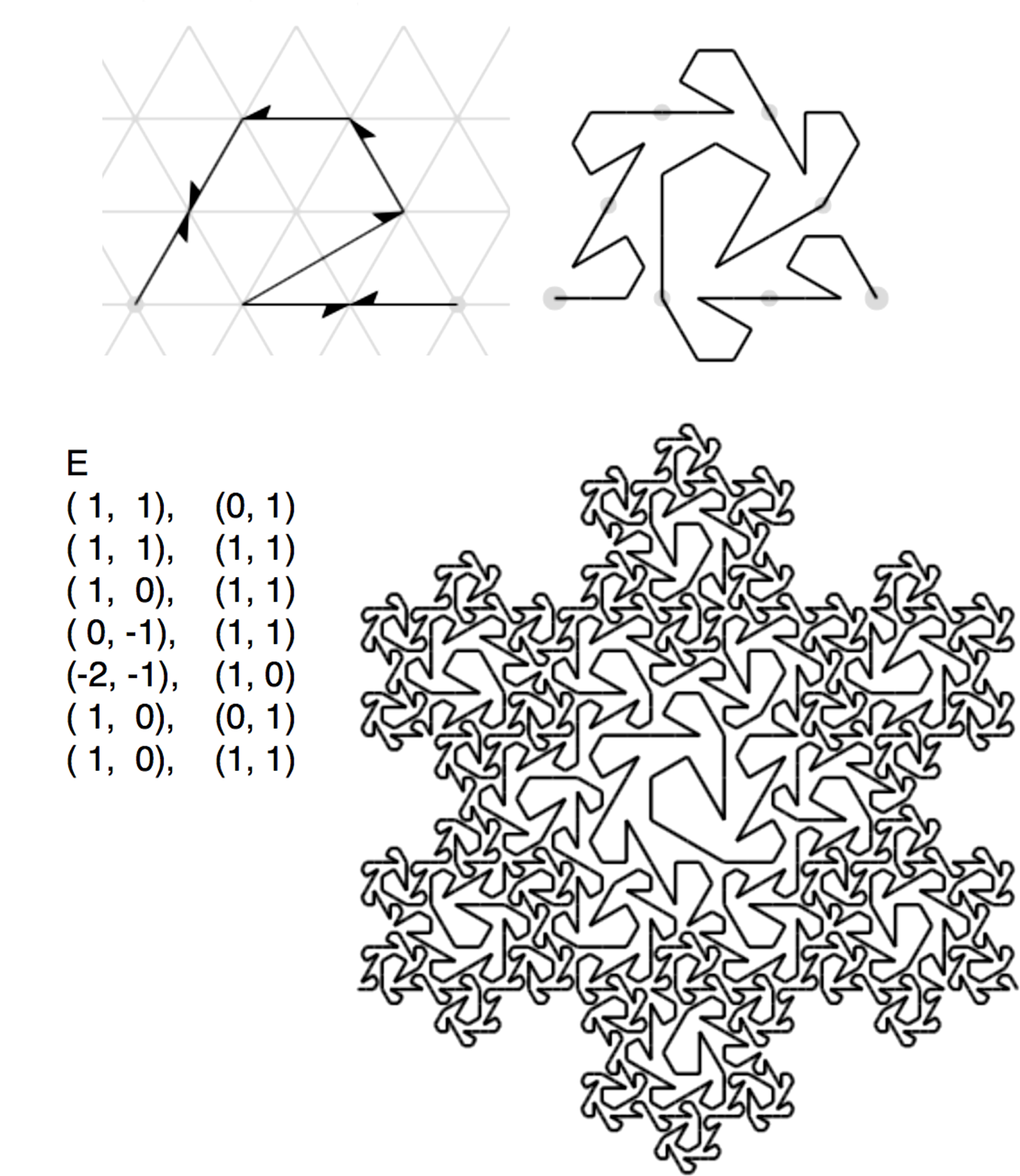Figure 3.12. The Snowflake sweep, showing minimal genetic encoding Search Space By reducing the information footprint of the genetic encoding in this way, it becomes easier to search the space of plane-filling curves, because the number of parameters that have to be considered are fewer (and thus, the number of dimensions in the search space). While searching for curves does require some brute-force computation—especially for larger families, the formulation of this taxonomy has made it much easier to discover the plane-filling curves shown in this book (with more featured in the website fractalcurves.com). Curves with fractal dimension < 2 Many of the now-familiar edge-replacement curves that were introduced by Mandelbrot can be described using this abbreviated genetic encoding. Not all of them are plane-filling, but since they obey the constraints of the integer lattice, they fit within this taxonomy. Figure 3.13 shows four examples: the Koch curve, the quadratic Koch curve, the Minkowski sausage, and the Monkey’s Tree. Since the first three example curves do not require any transforms, these are not included.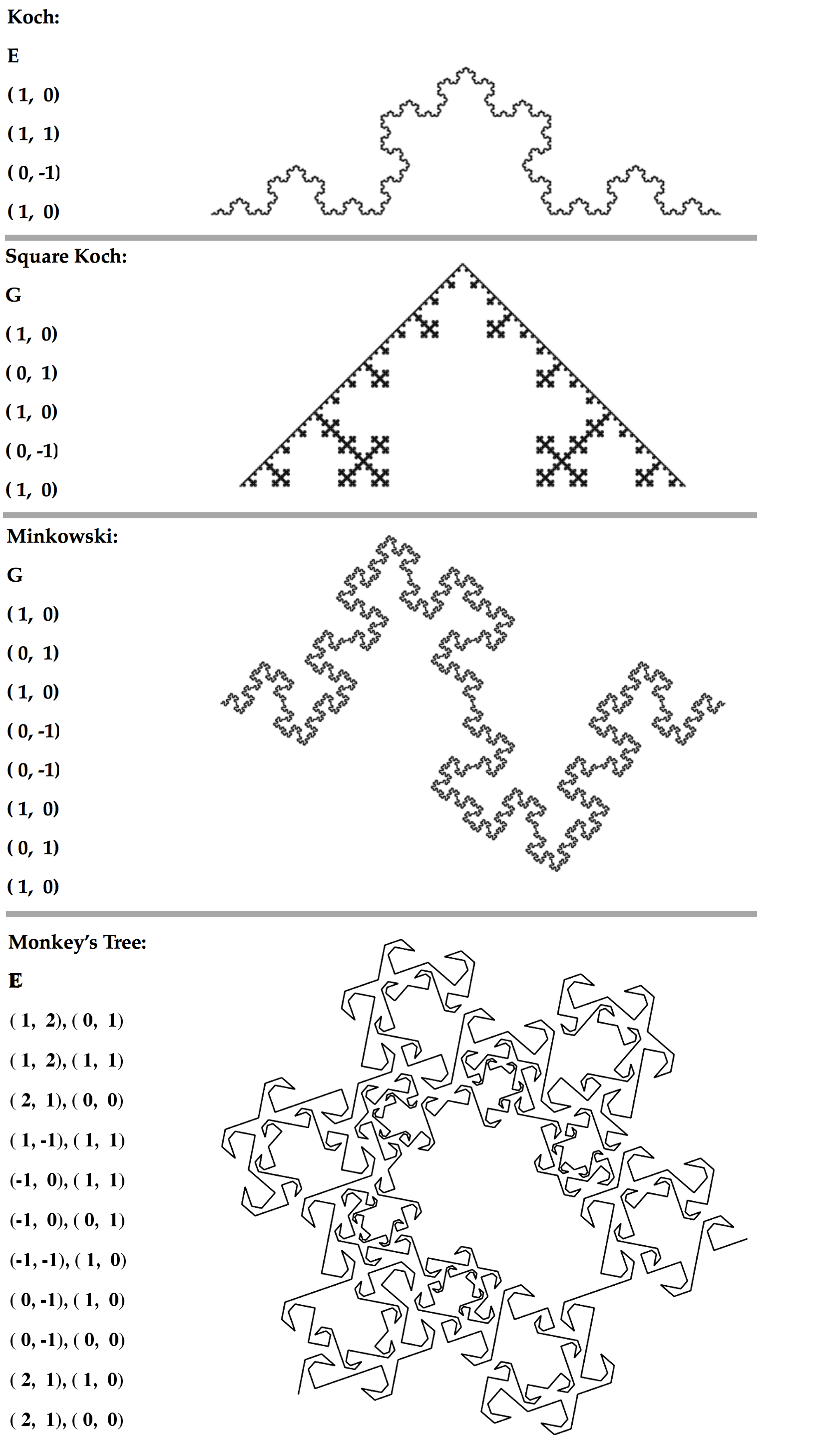Figure 3.13. Genetic encoding of curves with dimension < 2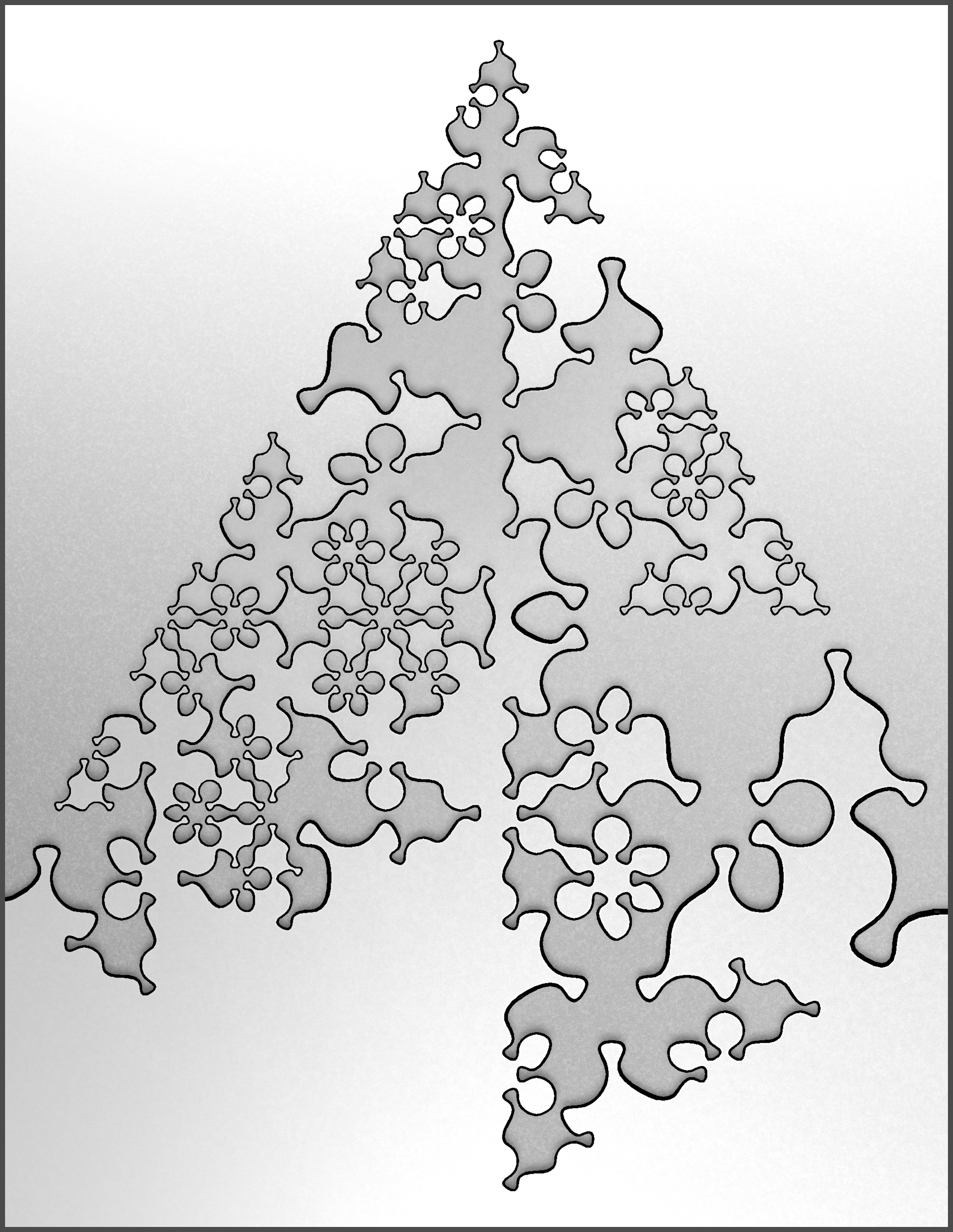Figure 3.14. A stylized rendering of a curve with dimension < 2 from the E(3,0) familyThe Family Tree of Fractal Curves A taxonomy of plane-filling curves using complex integer lattices by Jeffrey Ventrella 1 Introduction 2 Taxonomy 3 Iteration 4 Morphology 5 Family Resemblances 6 Undiscovered Curiosities 7 References